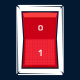# How to bypass WebGL Sop restriction v2

December 5th, 2011 by Henri

I’ve previously posted a trick to bypass the WebGL same origin policy restriction in a particular context. But my solution had a major drawback: it was slow due to jpeg decoding in javascript. Hopefully I’ve implemented a new solution which is way much faster thanks to John Bauman suggestion and it works like a charm!

```var xhr = new XMLHttpRequest();
xhr.open("GET", JPEG_URL, true);
xhr.responseType = "arraybuffer";
var img = new Image();
var tex = new THREE.Texture(this);
//do something with this texture...
};
img.src = arrayBufferDataUri(xhr.response);
};
xhr.send(null);
```

The function “arrayBufferDataUri” at the line 10 allow to speed-up the conversion from ArrayBuffer to base64 ascii string by avoiding an extra copy needed to use window.btoa. I’ve found this function here: http://jsperf.com/encoding-xhr-image-data/5.

```//From: http://jsperf.com/encoding-xhr-image-data/5
function arrayBufferDataUri(raw) {
var base64 = '';
var encodings = 'ABCDEFGHIJKLMNOPQRSTUVWXYZabcdefghijklmnopqrstuvwxyz0123456789+/';
var bytes = new Uint8Array(raw);
var byteLength = bytes.byteLength;
var byteRemainder = byteLength % 3;
var mainLength = byteLength - byteRemainder;
var a, b, c, d;
var chunk;
// Main loop deals with bytes in chunks of 3
for (var i = 0; i < mainLength; i = i + 3) {
// Combine the three bytes into a single integer
chunk = (bytes[i] << 16) | (bytes[i + 1] << 8 ) | bytes[i + 2];
// Use bitmasks to extract 6-bit segments from the triplet
a = (chunk & 16515072) >> 18; // 16515072 = (2^6 - 1) << 18
b = (chunk & 258048) >> 12;   // 258048   = (2^6 - 1) << 12
c = (chunk & 4032) >> 6;      // 4032     = (2^6 - 1) << 6
d = chunk & 63;               // 63       = 2^6 - 1
// Convert the raw binary segments to the appropriate ASCII encoding
base64 += encodings[a] + encodings[b] + encodings[c] + encodings[d]
}
// Deal with the remaining bytes and padding
if (byteRemainder == 1) {
chunk = bytes[mainLength];
a = (chunk & 252) >> 2; // 252 = (2^6 - 1) << 2
// Set the 4 least significant bits to zero
b = (chunk & 3) << 4;   // 3   = 2^2 - 1
base64 += encodings[a] + encodings[b] + '==';
}
else if (byteRemainder == 2) {
chunk = (bytes[mainLength] << 8 ) | bytes[mainLength + 1];
a = (chunk & 16128) >> 8; // 16128 = (2^6 - 1) << 8
b = (chunk & 1008) >> 4;  // 1008  = (2^6 - 1) << 4
// Set the 2 least significant bits to zero
c = (chunk & 15) << 2;    // 15    = 2^4 - 1
base64 += encodings[a] + encodings[b] + encodings[c] + '=';
}
return "data:image/jpeg;base64," + base64;
}
```

I’ve only used this code for my Google Chrome PhotoSynth WebGL extension. This is why I don’t have added extra check for other browser (line 10: xhr.mozResponseArrayBuffer || xhr.response).

1.Memmie Lenglet says:

Note: Now you can use Blobs instead of data URIs:
var blob = new Blob([xhr.response], {type : xhr.getResponseHeader(“Content-Type”) || ‘application/octet-binary’});
img.src = URL.createObjectURL(blob);

2.cheesyeyes says:

Hi!
Does this also work with video texture?
thanks!